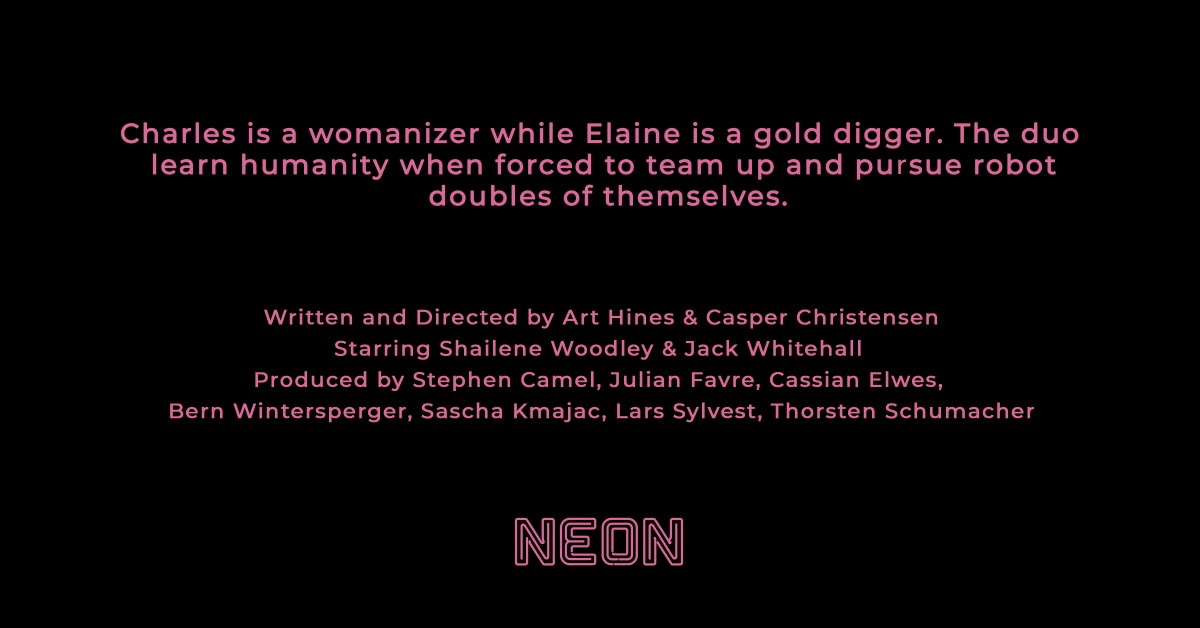## Official Trailer debut: ‘ROBOTS’ finds Jack Whitehall & Shailene Woodley at odds with “themselves”## 110 New Movies on HBO to Watch This Weekend![imdb id=”tt0453562″ plot=”short”]

[imdb id=”tt1272878″ plot=”short”]
[imdb id=”tt1335975″ plot=”short”]
[imdb id=”tt0298203″ plot=”short”]
[imdb id=”tt0305206″ plot=”short”]
[imdb id=”tt1816608″ plot=”short”]
[imdb id=”tt0118655″ plot=”short”]
[imdb id=”tt0109198″ plot=”short”]
[imdb id=”tt0159273″ plot=”short”]
[imdb id=”tt2083355″ plot=”short”]
[imdb id=”tt0094737″ plot=”short”]
[imdb id=”tt0077233″ plot=”short”]
[imdb id=”tt1425922″ plot=”short”]
[imdb id=”tt0181316″ plot=”short”]
[imdb id=”tt0452594″ plot=”short”]
[imdb id=”tt0074281″ plot=”short”]
[imdb id=”tt0824747″ plot=”short”]
[imdb id=”tt1438254″ plot=”short”]
[imdb id=”tt0115907″ plot=”short”]
[imdb id=”tt0120633″ plot=”short”]
[imdb id=”tt0142192″ plot=”short”]
[imdb id=”tt0142201″ plot=”short”]
[imdb id=”tt0112757″ plot=”short”]
[imdb id=”tt0104073″ plot=”short”]
[imdb id=”tt0361715″ plot=”short”]
[imdb id=”tt0262396″ plot=”short”]
[imdb id=”tt1211956″ plot=”short”]
[imdb id=”tt0356680″ plot=”short”]
[imdb id=”tt0297884″ plot=”short”]
[imdb id=”tt0408345″ plot=”short”]
[imdb id=”tt0116313″ plot=”short”]
[imdb id=”tt0418689″ plot=”short”]
[imdb id=”tt0910554″ plot=”short”]
[imdb id=”tt0144214″ plot=”short”]
[imdb id=”tt0123865″ plot=”short”]
[imdb id=”tt0172493″ plot=”short”]
[imdb id=”tt0433383″ plot=”short”]
[imdb id=”tt0401445″ plot=”short”]
[imdb id=”tt0456554″ plot=”short”]
[imdb id=”tt1454468″ plot=”short”]
[imdb id=”tt0104431″ plot=”short”]
[imdb id=”tt0120703″ plot=”short”]
[imdb id=”tt0373926″ plot=”short”]
[imdb id=”tt0057193″ plot=”short”]
[imdb id=”tt0120716″ plot=”short”]
[imdb id=”tt0102138″ plot=”short”]
[imdb id=”tt0116705″ plot=”short”]
[imdb id=”tt1583420″ plot=”short”]
[imdb id=”tt0455590″ plot=”short”]
[imdb id=”tt0097722″ plot=”short”]
[imdb id=”tt1043726″ plot=”short”]
[imdb id=”tt0084259″ plot=”short”]
[imdb id=”tt0199725″ plot=”short”]
[imdb id=”tt0120483″ plot=”short”]
[imdb id=”tt0056218″ plot=”short”]
[imdb id=”tt0433412″ plot=”short”]
[imdb id=”tt0100140″ plot=”short”]
[imdb id=”tt0095638″ plot=”short”]
[imdb id=”tt0104905″ plot=”short”]
[imdb id=”tt0246544″ plot=”short”]
[imdb id=”tt0382189″ plot=”short”]
[imdb id=”tt0113986″ plot=”short”]
[imdb id=”tt1670345″ plot=”short”]
[imdb id=”tt0084434″ plot=”short”]
[imdb id=”tt0100301″ plot=”short”]
[imdb id=”tt0119848″ plot=”short”]
[imdb id=”tt0490196″ plot=”short”]
[imdb id=”tt0107822″ plot=”short”]
[imdb id=”tt0098097″ plot=”short”]
[imdb id=”tt0119905″ plot=”short”]
[imdb id=”tt0081398″ plot=”short”]
[imdb id=”tt0105217″ plot=”short”]
[imdb id=”tt0078163″ plot=”short”]
[imdb id=”tt1408253″ plot=”short”]
[imdb id=”tt0267891″ plot=”short”]
[imdb id=”tt0358082″ plot=”short”]
[imdb id=”tt0073629″ plot=”short”]
[imdb id=”tt0120812″ plot=”short”]
[imdb id=”tt0195234″ plot=”short”]
[imdb id=”tt0257106″ plot=”short”]
[imdb id=”tt0081505″ plot=”short”]
[imdb id=”tt0284490″ plot=”short”]
[imdb id=”tt0158983″ plot=”short”]
[imdb id=”tt0120478″ plot=”short”]
[imdb id=”tt0157075″ plot=”short”]
[imdb id=”tt0090098″ plot=”short”]
[imdb id=”tt0094072″ plot=”short”]
[imdb id=”tt1161064″ plot=”short”]
[imdb id=”tt0167427″ plot=”short”]
[imdb id=”tt0427944″ plot=”short”]
[imdb id=”tt1800246″ plot=”short”]
[imdb id=”tt0094142″ plot=”short”]
[imdb id=”tt0220100″ plot=”short”]
[imdb id=”tt0084814″ plot=”short”]
[imdb id=”tt0120382″ plot=”short”]
[imdb id=”tt0100828″ plot=”short”]
[imdb id=”tt0118073″ plot=”short”]
[imdb id=”tt0100879″ plot=”short”]
[imdb id=”tt0086567″ plot=”short”]
[imdb id=”tt0317235″ plot=”short”]
[imdb id=”tt0120484″ plot=”short”]
[imdb id=”tt0055614″ plot=”short”]
[imdb id=”tt0181151″ plot=”short”]
[imdb id=”tt0327247″ plot=”short”]
[imdb id=”tt0324554″ plot=”short”]
[imdb id=”tt1837709″ plot=”short”]
[imdb id=”tt1430132″ plot=”short”]
[imdb id=”tt0120531″ plot=”short”]
[imdb id=”tt0096463″ plot=”short”]
[imdb id=”tt1213663″ plot=”short”]